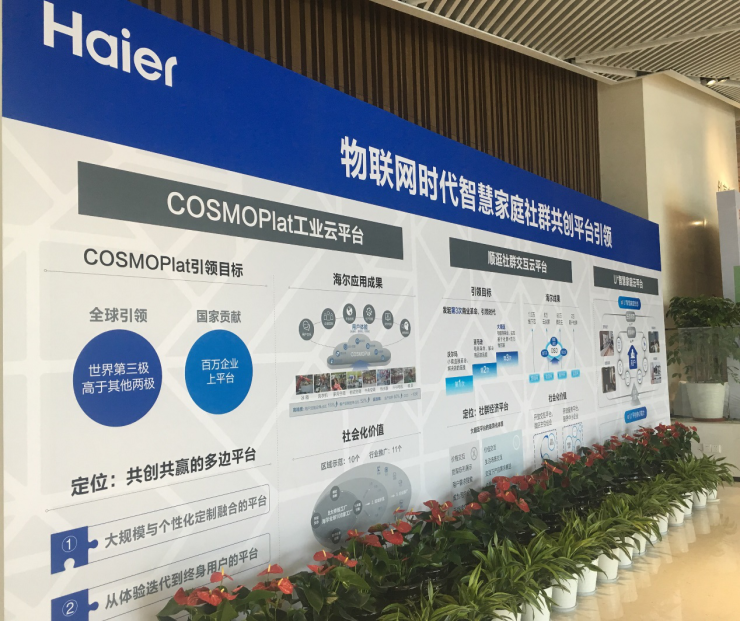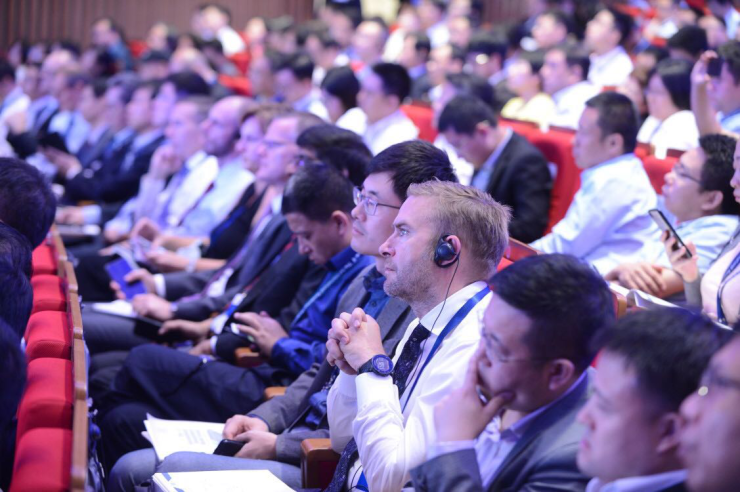|

# 海尔COSMOPlat打造世界第3种制造模式

海尔COSMOPlat打造世界第3种制造模式：以用户为中心

9月21日，海尔首届人单合一模式国际论坛迎来了全球管理模式领域“冰山论剑”的第二天。在人单合一模式引领下，海尔家电产业举办了以“智慧物联･社群共创”为主题的海尔智慧家庭全球引领创新峰会，在会上海尔向全球介绍了“人单合一”模式在制造业落地的首创——COSMOPlat，展现了继福特、丰田之后的第三种制造模式，实现了从以企业为中心向以用户为中心，从大规模制造到大规模定制的转型升级。从体验迭代到用户终身价值

随着传统制造业和互联网的快速融合，制造业也在发生巨大变化，原先由企业主导的大规模流水线生产逐渐开始向用户需求驱动下的大规模定制模式转型。而目前大部分企业对工业互联网的探索就是把生产线换成自动化大批量的生产，用机器取代人工。这样虽然可以增加生产线的灵活性和柔性，但是却无法实现用户对产品的个性化需求。因为，满足高度消费需求的制造体系才是未来中国制造业的特征，也是世界性的趋势。

作为中国最早探索智能制造的企业之一，海尔通过多年大规模定制的探索和实践，率先推出了以用户需求为核心驱动的工业互联网平台——COSMOPlat，平台可以让用户参与到体验的全流程，实现由顾客到交互用户最终成为终身用户，同时帮助企业实现产品价值到生态价值。以用户需求为驱动，通过规模化生产和高效供应链管理，实现了与用户的“零距离”，破解了个性化定制与大规模之间的矛盾，这在全球的制造模式中不仅是首次也是唯一。以贝享空调为例，在母婴社群，有很多妈妈抱怨目前市面上的空调噪音大等，针对用户的这些痛点，在COSMOPlat上很快得到在线资源的响应，设计资源和模块商资源参与进来和用户进行在线交互。同时，针对用户提出的7大产品功能需求，经过5次方案迭代，在今年的5月份迭代出了满足用户静音功能的静+空调，实现行业最低噪音17分贝，首次预售便突破1.1万台。

目前，在海尔COSMOPlat的运作之下，海尔已经打造起沈阳冰箱、胶州空调、青岛中央空调等8大互联工厂，生产效率提升60%，产品的不入库率达到了68%。现在，海尔已经将互联工厂样板向全球108个工厂推广，从而实现在全球范围内的大规模定制模式。

物联网时代下共创共赢的多边平台

“中国制造2025”根本目标在于改变中国制造业"大而不强"的局面，通过10年努力使中国迈入制造强国行列，为到2045年将中国建成具有全球引领和影响力的制造强国奠定坚实基础。具体在重点领域推进智能制造、大规模个性化定制、网络化协同制造和服务型制造，打造一批网络化协同制造公共服务平台，加快形成制造业网络化产业生态体系。

海尔把多年经验产品化、微服务化，构建了面向全球、全行业的工业大规模定制平台COSMOPlat，而且COSMOPlat不仅服务于家电行业，同样可以扩展至家居、服装等行业。目前，COSMOPlat平台服务已拓展至山东、上海天津等10大区域，在服装、农业、汽车、化工等11个行业实现了“复制”。以服装行业为例，服装行业是个亿万级市场，目前服装行业存在两大痛点：库存重和消费者偏好追踪难。而COSMOPlat将制衣环节及物流配送环节数据采集并沉淀到平台上，并通过算法和模型进行渠道物流的精细化管理，解决了库存问题；同时通过社群交互挖掘用户痛点和创意，对用户需求及流行款式进行预测。

在亟需产业结构调整的时刻，海尔COSMOPlat成功落地并实践，为我国工业制造带来了机遇和希望。COSMOPlat也凭借其开放和共享的平台，入选了工信部首批服务型制造示范项目、2017年制造业与互联网融合发展试点示范项目，此外，在全球权威机构高德纳评选的“高德纳2017高科技制造创新者奖”中，COSMOPlat成为亚太地区唯一获奖企业，也是全球工业制造业第一个、目前唯一一个获得该奖项的企业。

在当前新一轮工业革命一触即发之际，海尔COSMOPlat平台通过“多快好省”的聚合能力使资源方和用户蜂拥而至、为他们提供数据类、应用流程和模式服务。同时 COSMOPlat平台通过母平台使能、子平台拓展的战略为各区域、各行业提供一个转型升级的世界级平台，代表中国制造走在了全球制造业转型升级的前列。

`声明：本文由入驻焦点开放平台的作者撰写，除焦点官方账号外，观点仅代表作者本人，不代表焦点立场错误信息举报电话： 400-099-0099，邮箱：jubao@vip.sohu.com，或点此进行意见反馈，或点此进行举报投诉。`A B C D E F G H J K L M N P Q R S T W X Y Z
A - B - C - D - E
• A
• 鞍山
• 安庆
• 安阳
• 安顺
• 安康
• 澳门
• B
• 北京
• 保定
• 包头
• 巴彦淖尔
• 本溪
• 蚌埠
• 亳州
• 滨州
• 北海
• 百色
• 巴中
• 毕节
• 保山
• 宝鸡
• 白银
• 巴州
• C
• 承德
• 沧州
• 长治
• 赤峰
• 朝阳
• 长春
• 常州
• 滁州
• 池州
• 长沙
• 常德
• 郴州
• 潮州
• 崇左
• 重庆
• 成都
• 楚雄
• 昌都
• 慈溪
• 常熟
• D
• 大同
• 大连
• 丹东
• 大庆
• 东营
• 德州
• 东莞
• 德阳
• 达州
• 大理
• 德宏
• 定西
• 儋州
• 东平
• E
• 鄂尔多斯
• 鄂州
• 恩施
F - G - H - I - J
• F
• 抚顺
• 阜新
• 阜阳
• 福州
• 抚州
• 佛山
• 防城港
• G
• 赣州
• 广州
• 桂林
• 贵港
• 广元
• 广安
• 贵阳
• 固原
• H
• 邯郸
• 衡水
• 呼和浩特
• 呼伦贝尔
• 葫芦岛
• 哈尔滨
• 黑河
• 淮安
• 杭州
• 湖州
• 合肥
• 淮南
• 淮北
• 黄山
• 菏泽
• 鹤壁
• 黄石
• 黄冈
• 衡阳
• 怀化
• 惠州
• 河源
• 贺州
• 河池
• 海口
• 红河
• 汉中
• 海东
• I
• J
• 晋中
• 锦州
• 吉林
• 鸡西
• 佳木斯
• 嘉兴
• 金华
• 景德镇
• 九江
• 吉安
• 济南
• 济宁
• 焦作
• 荆门
• 荆州
• 江门
• 揭阳
• 金昌
• 酒泉
• 嘉峪关
K - L - M - N - P
• K
• 开封
• 昆明
• 昆山
• L
• 廊坊
• 临汾
• 辽阳
• 连云港
• 丽水
• 六安
• 龙岩
• 莱芜
• 临沂
• 聊城
• 洛阳
• 漯河
• 娄底
• 柳州
• 来宾
• 泸州
• 乐山
• 六盘水
• 丽江
• 临沧
• 拉萨
• 林芝
• 兰州
• 陇南
• M
• 牡丹江
• 马鞍山
• 茂名
• 梅州
• 绵阳
• 眉山
• N
• 南京
• 南通
• 宁波
• 南平
• 宁德
• 南昌
• 南阳
• 南宁
• 内江
• 南充
• P
• 盘锦
• 莆田
• 平顶山
• 濮阳
• 攀枝花
• 普洱
• 平凉
Q - R - S - T - W
• Q
• 秦皇岛
• 齐齐哈尔
• 衢州
• 泉州
• 青岛
• 清远
• 钦州
• 黔南
• 曲靖
• 庆阳
• R
• 日照
• 日喀则
• S
• 石家庄
• 沈阳
• 双鸭山
• 绥化
• 上海
• 苏州
• 宿迁
• 绍兴
• 宿州
• 三明
• 上饶
• 三门峡
• 商丘
• 十堰
• 随州
• 邵阳
• 韶关
• 深圳
• 汕头
• 汕尾
• 三亚
• 三沙
• 遂宁
• 山南
• 商洛
• 石嘴山
• T
• 天津
• 唐山
• 太原
• 通辽
• 铁岭
• 泰州
• 台州
• 铜陵
• 泰安
• 铜仁
• 铜川
• 天水
• 天门
• W
• 乌海
• 乌兰察布
• 无锡
• 温州
• 芜湖
• 潍坊
• 威海
• 武汉
• 梧州
• 渭南
• 武威
• 吴忠
• 乌鲁木齐
X - Y - Z
• X
• 邢台
• 徐州
• 宣城
• 厦门
• 新乡
• 许昌
• 信阳
• 襄阳
• 孝感
• 咸宁
• 湘潭
• 湘西
• 西双版纳
• 西安
• 咸阳
• 西宁
• 仙桃
• 西昌
• Y
• 运城
• 营口
• 盐城
• 扬州
• 鹰潭
• 宜春
• 烟台
• 宜昌
• 岳阳
• 益阳
• 永州
• 阳江
• 云浮
• 玉林
• 宜宾
• 雅安
• 玉溪
• 延安
• 榆林
• 银川
• Z
• 张家口
• 镇江
• 舟山
• 漳州
• 淄博
• 枣庄
• 郑州
• 周口
• 驻马店
• 株洲
• 张家界
• 珠海
• 湛江
• 肇庆
• 中山
• 自贡
• 资阳
• 遵义
• 昭通
• 张掖
• 中卫

1室1厅1厨1卫1阳台

1
2
3
4
5

0
1
2

1

1

0
1
2
3报名成功，资料已提交审核A B C D E F G H J K L M N P Q R S T W X Y Z
A - B - C - D - E
• A
• 鞍山
• 安庆
• 安阳
• 安顺
• 安康
• 澳门
• B
• 北京
• 保定
• 包头
• 巴彦淖尔
• 本溪
• 蚌埠
• 亳州
• 滨州
• 北海
• 百色
• 巴中
• 毕节
• 保山
• 宝鸡
• 白银
• 巴州
• C
• 承德
• 沧州
• 长治
• 赤峰
• 朝阳
• 长春
• 常州
• 滁州
• 池州
• 长沙
• 常德
• 郴州
• 潮州
• 崇左
• 重庆
• 成都
• 楚雄
• 昌都
• 慈溪
• 常熟
• D
• 大同
• 大连
• 丹东
• 大庆
• 东营
• 德州
• 东莞
• 德阳
• 达州
• 大理
• 德宏
• 定西
• 儋州
• 东平
• E
• 鄂尔多斯
• 鄂州
• 恩施
F - G - H - I - J
• F
• 抚顺
• 阜新
• 阜阳
• 福州
• 抚州
• 佛山
• 防城港
• G
• 赣州
• 广州
• 桂林
• 贵港
• 广元
• 广安
• 贵阳
• 固原
• H
• 邯郸
• 衡水
• 呼和浩特
• 呼伦贝尔
• 葫芦岛
• 哈尔滨
• 黑河
• 淮安
• 杭州
• 湖州
• 合肥
• 淮南
• 淮北
• 黄山
• 菏泽
• 鹤壁
• 黄石
• 黄冈
• 衡阳
• 怀化
• 惠州
• 河源
• 贺州
• 河池
• 海口
• 红河
• 汉中
• 海东
• I
• J
• 晋中
• 锦州
• 吉林
• 鸡西
• 佳木斯
• 嘉兴
• 金华
• 景德镇
• 九江
• 吉安
• 济南
• 济宁
• 焦作
• 荆门
• 荆州
• 江门
• 揭阳
• 金昌
• 酒泉
• 嘉峪关
K - L - M - N - P
• K
• 开封
• 昆明
• 昆山
• L
• 廊坊
• 临汾
• 辽阳
• 连云港
• 丽水
• 六安
• 龙岩
• 莱芜
• 临沂
• 聊城
• 洛阳
• 漯河
• 娄底
• 柳州
• 来宾
• 泸州
• 乐山
• 六盘水
• 丽江
• 临沧
• 拉萨
• 林芝
• 兰州
• 陇南
• M
• 牡丹江
• 马鞍山
• 茂名
• 梅州
• 绵阳
• 眉山
• N
• 南京
• 南通
• 宁波
• 南平
• 宁德
• 南昌
• 南阳
• 南宁
• 内江
• 南充
• P
• 盘锦
• 莆田
• 平顶山
• 濮阳
• 攀枝花
• 普洱
• 平凉
Q - R - S - T - W
• Q
• 秦皇岛
• 齐齐哈尔
• 衢州
• 泉州
• 青岛
• 清远
• 钦州
• 黔南
• 曲靖
• 庆阳
• R
• 日照
• 日喀则
• S
• 石家庄
• 沈阳
• 双鸭山
• 绥化
• 上海
• 苏州
• 宿迁
• 绍兴
• 宿州
• 三明
• 上饶
• 三门峡
• 商丘
• 十堰
• 随州
• 邵阳
• 韶关
• 深圳
• 汕头
• 汕尾
• 三亚
• 三沙
• 遂宁
• 山南
• 商洛
• 石嘴山
• T
• 天津
• 唐山
• 太原
• 通辽
• 铁岭
• 泰州
• 台州
• 铜陵
• 泰安
• 铜仁
• 铜川
• 天水
• 天门
• W
• 乌海
• 乌兰察布
• 无锡
• 温州
• 芜湖
• 潍坊
• 威海
• 武汉
• 梧州
• 渭南
• 武威
• 吴忠
• 乌鲁木齐
X - Y - Z
• X
• 邢台
• 徐州
• 宣城
• 厦门
• 新乡
• 许昌
• 信阳
• 襄阳
• 孝感
• 咸宁
• 湘潭
• 湘西
• 西双版纳
• 西安
• 咸阳
• 西宁
• 仙桃
• 西昌
• Y
• 运城
• 营口
• 盐城
• 扬州
• 鹰潭
• 宜春
• 烟台
• 宜昌
• 岳阳
• 益阳
• 永州
• 阳江
• 云浮
• 玉林
• 宜宾
• 雅安
• 玉溪
• 延安
• 榆林
• 银川
• Z
• 张家口
• 镇江
• 舟山
• 漳州
• 淄博
• 枣庄
• 郑州
• 周口
• 驻马店
• 株洲
• 张家界
• 珠海
• 湛江
• 肇庆
• 中山
• 自贡
• 资阳
• 遵义
• 昭通
• 张掖
• 中卫• 手机• 分享
• 设计
免费设计
• 计算器
装修计算器
• 入驻
合作入驻
• 联系
联系我们
• 置顶
返回顶部